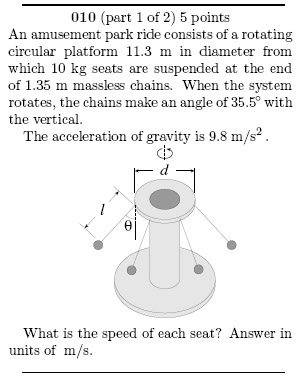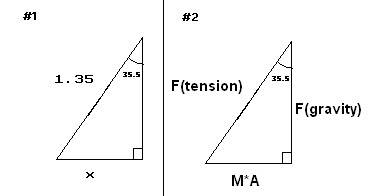# IB 11 Question: Circular Acceleration Problem Involving Forces

Hi, this question is a part of an 'online homework packet I recieved through my IB 11 SL course (ie. International Baccalaureate 11 Standard Leval). I submitted my first attempt to answer this question and had the wonderful "sorry your answer is incorrect" screen pop up. I was wondering if I could get some advice on howe to solve the question. Am I understanding the concept but doing something wrong when it comes to calculations? Or do I need a push in the right direction with concept?
ThanksJL

1.2.• (Total Force) = mass * acceleration
• (Centrepital Acceleration) = (Velocity squared) / (Radius)
• (Force of Gravity) = (mass)*(9.8)

3.
• Using trig. Radius = (sin35.5 *1.35) + (diameter/2) =6.43394899 m
• (Force of Gravity) = 9.8 * 10 kg = 98 N
• Using trig (Total Force) = mass*acceleration = (tan 35.5 *Force of Gravity)= 69.990272065
• (Acceleration) = (Total Force)/mass = 69.990272065/10 =6.9990272065 m/s^2

I first tried to calculate the radius adding the bottom part of the first triangle to half of the diameter.I'm fairly certain of the radius but if anyone thinks up another method or thinks that this method is incorrect please let me know.
I secondly calculated the Force of gravity on the 10 kg seat
Using the second triangle I used trig to solve for the total force
I then substituted the Total force (ie. Mass * Acceleration) and mass to solve for Acceleration.

## The Attempt at a Solution

As you can all pretty much see that was my first post, if I'm doing anything wrong, (asking the wrong kind of question, not clear, whatever it is) let me know, thx

andrevdh
Homework Helper
Everything seems to be fine. Although you could have made life a bit easier for yourself:

$$m a = m g \tan(\theta)$$

$$\frac{v^2}{r} = g \tan(\theta)$$

What value do you then get for the speed?

Last edited:
actually sumone in my class told me that i should have used 90(degrees) minus theta in my second triangle , it works but i don't know why?!?!

wait no i got it, my bad it was a calculation error!andrevdh
Homework Helper
?????????????????